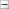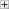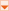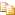﻿iCalendarCollection MethodsThe iCalendarCollection type exposes the following members.

#Methods

NameDescriptionAdd
Adds an object to the end of the List<(Of <(<'T>)>)>.
(Inherited from List<(Of <(<'IICalendar>)>)>.)AddRange
Adds the elements of the specified collection to the end of the List<(Of <(<'T>)>)>.
(Inherited from List<(Of <(<'IICalendar>)>)>.)AsReadOnly
Returns a read-only IList<(Of <(<'T>)>)> wrapper for the current collection.
(Inherited from List<(Of <(<'IICalendar>)>)>.)BinarySearch(T)
Searches the entire sorted List<(Of <(<'T>)>)> for an element using the default comparer and returns the zero-based index of the element.
(Inherited from List<(Of <(<'IICalendar>)>)>.)BinarySearch(T, IComparer<(Of <<'(T>)>>))
Searches the entire sorted List<(Of <(<'T>)>)> for an element using the specified comparer and returns the zero-based index of the element.
(Inherited from List<(Of <(<'IICalendar>)>)>.)BinarySearch(Int32, Int32, T, IComparer<(Of <<'(T>)>>))
Searches a range of elements in the sorted List<(Of <(<'T>)>)> for an element using the specified comparer and returns the zero-based index of the element.
(Inherited from List<(Of <(<'IICalendar>)>)>.)Clear
Removes all elements from the List<(Of <(<'T>)>)>.
(Inherited from List<(Of <(<'IICalendar>)>)>.)ClearEvaluationContains
Determines whether an element is in the List<(Of <(<'T>)>)>.
(Inherited from List<(Of <(<'IICalendar>)>)>.)ConvertAll<(Of <<'(TOutput>)>>)
Converts the elements in the current List<(Of <(<'T>)>)> to another type, and returns a list containing the converted elements.
(Inherited from List<(Of <(<'IICalendar>)>)>.)CopyTo(array<T>[]()[][])
Copies the entire List<(Of <(<'T>)>)> to a compatible one-dimensional array, starting at the beginning of the target array.
(Inherited from List<(Of <(<'IICalendar>)>)>.)CopyTo(array<T>[]()[][], Int32)
Copies the entire List<(Of <(<'T>)>)> to a compatible one-dimensional array, starting at the specified index of the target array.
(Inherited from List<(Of <(<'IICalendar>)>)>.)CopyTo(Int32, array<T>[]()[][], Int32, Int32)
Copies a range of elements from the List<(Of <(<'T>)>)> to a compatible one-dimensional array, starting at the specified index of the target array.
(Inherited from List<(Of <(<'IICalendar>)>)>.)Equals
Determines whether the specified Object is equal to the current Object.
(Inherited from Object.)Exists
Determines whether the List<(Of <(<'T>)>)> contains elements that match the conditions defined by the specified predicate.
(Inherited from List<(Of <(<'IICalendar>)>)>.)Finalize
Allows an Object to attempt to free resources and perform other cleanup operations before the Object is reclaimed by garbage collection.
(Inherited from Object.)Find
Searches for an element that matches the conditions defined by the specified predicate, and returns the first occurrence within the entire List<(Of <(<'T>)>)>.
(Inherited from List<(Of <(<'IICalendar>)>)>.)FindAll
Retrieves all the elements that match the conditions defined by the specified predicate.
(Inherited from List<(Of <(<'IICalendar>)>)>.)FindIndex(Predicate<(Of <<'(T>)>>))
Searches for an element that matches the conditions defined by the specified predicate, and returns the zero-based index of the first occurrence within the entire List<(Of <(<'T>)>)>.
(Inherited from List<(Of <(<'IICalendar>)>)>.)FindIndex(Int32, Predicate<(Of <<'(T>)>>))
Searches for an element that matches the conditions defined by the specified predicate, and returns the zero-based index of the first occurrence within the range of elements in the List<(Of <(<'T>)>)> that extends from the specified index to the last element.
(Inherited from List<(Of <(<'IICalendar>)>)>.)FindIndex(Int32, Int32, Predicate<(Of <<'(T>)>>))
Searches for an element that matches the conditions defined by the specified predicate, and returns the zero-based index of the first occurrence within the range of elements in the List<(Of <(<'T>)>)> that starts at the specified index and contains the specified number of elements.
(Inherited from List<(Of <(<'IICalendar>)>)>.)FindLast
Searches for an element that matches the conditions defined by the specified predicate, and returns the last occurrence within the entire List<(Of <(<'T>)>)>.
(Inherited from List<(Of <(<'IICalendar>)>)>.)FindLastIndex(Predicate<(Of <<'(T>)>>))
Searches for an element that matches the conditions defined by the specified predicate, and returns the zero-based index of the last occurrence within the entire List<(Of <(<'T>)>)>.
(Inherited from List<(Of <(<'IICalendar>)>)>.)FindLastIndex(Int32, Predicate<(Of <<'(T>)>>))
Searches for an element that matches the conditions defined by the specified predicate, and returns the zero-based index of the last occurrence within the range of elements in the List<(Of <(<'T>)>)> that extends from the first element to the specified index.
(Inherited from List<(Of <(<'IICalendar>)>)>.)FindLastIndex(Int32, Int32, Predicate<(Of <<'(T>)>>))
Searches for an element that matches the conditions defined by the specified predicate, and returns the zero-based index of the last occurrence within the range of elements in the List<(Of <(<'T>)>)> that contains the specified number of elements and ends at the specified index.
(Inherited from List<(Of <(<'IICalendar>)>)>.)ForEach
Performs the specified action on each element of the List<(Of <(<'T>)>)>.
(Inherited from List<(Of <(<'IICalendar>)>)>.)GetEnumerator
Returns an enumerator that iterates through the List<(Of <(<'T>)>)>.
(Inherited from List<(Of <(<'IICalendar>)>)>.)GetHashCode
Serves as a hash function for a particular type.
(Inherited from Object.)GetOccurrences(DateTime)GetOccurrences(IDateTime)GetOccurrences(DateTime, DateTime)GetOccurrences(IDateTime, IDateTime)GetOccurrences<(Of <<'(T>)>>)(DateTime)GetOccurrences<(Of <<'(T>)>>)(IDateTime)GetOccurrences<(Of <<'(T>)>>)(DateTime, DateTime)GetOccurrences<(Of <<'(T>)>>)(IDateTime, IDateTime)GetRange
Creates a shallow copy of a range of elements in the source List<(Of <(<'T>)>)>.
(Inherited from List<(Of <(<'IICalendar>)>)>.)GetType
Gets the Type of the current instance.
(Inherited from Object.)IndexOf(T)
Searches for the specified object and returns the zero-based index of the first occurrence within the entire List<(Of <(<'T>)>)>.
(Inherited from List<(Of <(<'IICalendar>)>)>.)IndexOf(T, Int32)
Searches for the specified object and returns the zero-based index of the first occurrence within the range of elements in the List<(Of <(<'T>)>)> that extends from the specified index to the last element.
(Inherited from List<(Of <(<'IICalendar>)>)>.)IndexOf(T, Int32, Int32)
Searches for the specified object and returns the zero-based index of the first occurrence within the range of elements in the List<(Of <(<'T>)>)> that starts at the specified index and contains the specified number of elements.
(Inherited from List<(Of <(<'IICalendar>)>)>.)Insert
Inserts an element into the List<(Of <(<'T>)>)> at the specified index.
(Inherited from List<(Of <(<'IICalendar>)>)>.)InsertRange
Inserts the elements of a collection into the List<(Of <(<'T>)>)> at the specified index.
(Inherited from List<(Of <(<'IICalendar>)>)>.)LastIndexOf(T)
Searches for the specified object and returns the zero-based index of the last occurrence within the entire List<(Of <(<'T>)>)>.
(Inherited from List<(Of <(<'IICalendar>)>)>.)LastIndexOf(T, Int32)
Searches for the specified object and returns the zero-based index of the last occurrence within the range of elements in the List<(Of <(<'T>)>)> that extends from the first element to the specified index.
(Inherited from List<(Of <(<'IICalendar>)>)>.)LastIndexOf(T, Int32, Int32)
Searches for the specified object and returns the zero-based index of the last occurrence within the range of elements in the List<(Of <(<'T>)>)> that contains the specified number of elements and ends at the specified index.
(Inherited from List<(Of <(<'IICalendar>)>)>.)MemberwiseClone
Creates a shallow copy of the current Object.
(Inherited from Object.)Remove
Removes the first occurrence of a specific object from the List<(Of <(<'T>)>)>.
(Inherited from List<(Of <(<'IICalendar>)>)>.)RemoveAll
Removes the all the elements that match the conditions defined by the specified predicate.
(Inherited from List<(Of <(<'IICalendar>)>)>.)RemoveAt
Removes the element at the specified index of the List<(Of <(<'T>)>)>.
(Inherited from List<(Of <(<'IICalendar>)>)>.)RemoveRange
Removes a range of elements from the List<(Of <(<'T>)>)>.
(Inherited from List<(Of <(<'IICalendar>)>)>.)Reverse()()()()
Reverses the order of the elements in the entire List<(Of <(<'T>)>)>.
(Inherited from List<(Of <(<'IICalendar>)>)>.)Reverse(Int32, Int32)
Reverses the order of the elements in the specified range.
(Inherited from List<(Of <(<'IICalendar>)>)>.)Sort()()()()
Sorts the elements in the entire List<(Of <(<'T>)>)> using the default comparer.
(Inherited from List<(Of <(<'IICalendar>)>)>.)Sort(IComparer<(Of <<'(T>)>>))
Sorts the elements in the entire List<(Of <(<'T>)>)> using the specified comparer.
(Inherited from List<(Of <(<'IICalendar>)>)>.)Sort(Comparison<(Of <<'(T>)>>))
Sorts the elements in the entire List<(Of <(<'T>)>)> using the specified Comparison<(Of <(<'T>)>)>.
(Inherited from List<(Of <(<'IICalendar>)>)>.)Sort(Int32, Int32, IComparer<(Of <<'(T>)>>))
Sorts the elements in a range of elements in List<(Of <(<'T>)>)> using the specified comparer.
(Inherited from List<(Of <(<'IICalendar>)>)>.)ToArray
Copies the elements of the List<(Of <(<'T>)>)> to a new array.
(Inherited from List<(Of <(<'IICalendar>)>)>.)ToString
Returns a String that represents the current Object.
(Inherited from Object.)TrimExcess
Sets the capacity to the actual number of elements in the List<(Of <(<'T>)>)>, if that number is less than a threshold value.
(Inherited from List<(Of <(<'IICalendar>)>)>.)TrueForAll
Determines whether every element in the List<(Of <(<'T>)>)> matches the conditions defined by the specified predicate.
(Inherited from List<(Of <(<'IICalendar>)>)>.)